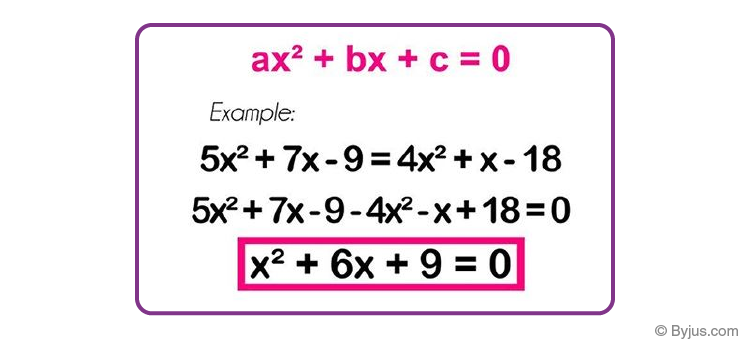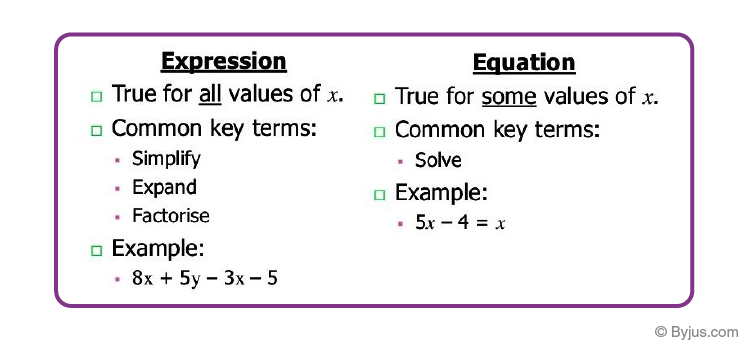# Algebraic Equations

An algebraic equation can be defined as a mathematical statement in which two expressions are set equal to each other. The algebraic equation usually consists of a variable, coefficients and constants.

Also Check: Algebraic Expressions.

In simple words, equations mean equality i.e. the equal sign. That’s what equations are all about- “equating one quantity with another”.Algebraic Equations

Equations are like a balance scale. If you’ve seen a balance scale, you would know that an equal amount of weight has to be placed on either side for the scale to be considered “balanced”. If we add some weight to just one side, the scale will tip on one side and the two sides are no longer in balance. Equations follow the same logic. Whatever is on one side of the equal sign must have exactly the same value on the other side else it becomes an inequality.

## What are Algebraic Equations?

An algebraic equation is an equation in the form:

P = 0

Where P is a polynomial.

For example, x + 8 = 0 is an algebraic equation, where x + 8 is a polynomial. Hence, it is also called a polynomial equation.

An algebraic equation is always a balanced equation that includes variables, coefficients, and constants.

Consider an equation 1+1 = 2.

It is balanced as both sides have the same value. To avoid committing an error that tips the equation out of balance, make sure that any change on one side of the equation is reciprocated on the other side. For example, if you want to add a number 5 to one side of the equation you will have to add the same 5 to the other side of the equation i.e.

1 + 1 = 2

1 + 1 + 5 = 2 + 5

The same goes for subtraction, multiplication, and division. As long as you do the same thing to both sides of the equation it will remain balanced.

## What is an Equation?

An equation is simply defined as mathematical statements that express the relationship between two values. Usually, the two values are equated by an equal sign in an equation.

For example, 2x+3 = 7 is an equation, where 2x+3 and 7 are equated by equal to “=” sign.

2x+3 is at the Left-hand side of the equation and 7 is at the right-hand side. In this example,

• 2x, 3 and 7 are terms
• x is the variable
• 3 and 7 are the constants
• ‘+’ is the operator

If we write x = 3, then it is also an equation, where we are denoting the value of variable x equal to 3.

## Types of Algebraic Equations

Algebraic equations are of various types. A few of the equations in algebra are:

• Polynomial Equations
• Cubic Equations
• Rational polynomial Equations
• Trigonometric Equations

### Polynomial Equations

All the polynomial equations are a part of algebraic equations like the linear equations. To recall, a polynomial equation is an equation consisting of variables, exponents and coefficients.

• Linear equations: ax+b=c (a not equal to 0)

A quadratic equation is a polynomial equation of degree 2 in one variable of type f(x) = ax2 + bx + c.

• Quadratic Equations: ax2+bx+c=0 (a not equal to 0)

### Cubic Equations

The cubic polynomials are polynomials with degree 3. All the cubic polynomials are also algebraic equations.

• Cubic Polynomials: ax3+bx2+cx+d=0

• P(x)/Q(x)=0

### Trigonometric Equations

All the trigonometric equations are all considered as algebraic functions. For a trigonometry equation, the expression includes the trigonometric functions of a variable.

• Trigonometric Equations: cos2x = 1+4sinx

## How to Solve Algebraic Equations

Consider the following situation. I am going on a trip. In one bag I carry some t-shirts, shorts, and towels. A total of 8 items can fit in the bag. So I pack 4 shirts and 2 shorts. How many towels can I now carry?

Consider the number of towels to be ‘x’. Let’s form the equation now.

4 shirts + 2 shorts + ‘x’ towels = 8 clothes

The left-hand side (LHS) of our equation is being compared to the right-hand side (RHS) of the equation.

Now, let’s solve this equation:

4+2+x=8

6+x=8

6+x-6=8−6

x=2

I can carry 2 towels for my trip.

In the same way, what would depict an inequality? Obviously, when the left-hand side is not equal to the right-hand side. How would this happen?

Let’s take the same 6 + x = 8 and change that equal to into a greater than or a lesser than sign. These aren’t equations! Consider some examples to clarify this concept.

x + 2 = 21, xy + 9 = z are equations but 6p > 77 is not.

## Solved Examples

Q.1: Simplify the given equation : 2(x+4)+3(x–5)–2y=0

Solution:

Given equation: 2(x+4)+3(x−5)–2y=0

2x+2×4+3x–3×5–2y=0 (Using Distributive property to get rid of parenthesis)

2x+8+3x–15–2y=0 (Simplifying)

5x–2y–7=0 (on further simplifying terms)

Q.2: Solve x + 1 = 9

Solution: Given, x+1 = 9

If we bring 1 from LHS to RHS, then the sign of 1 will get change.

x = 9 – 1

x = 8

Hence, is the solution.

Q.3: Solve 15 + 5x = 0.

Solution: Given, 15 + 5x = 0

We need to solve for x here.

Let the term with x remain on the LHS and move the rest terms to the RHS.

5x = 0 – 15

5x = -15

Now divide both the sides by 5.

(5x)/5 = -15/5

x = -3

Thus, x = -3 is the required solution.

Q.4: Solve -10x – 19 = 19 – 8x

Solution: Given,-10x – 19 = 19 – 8x is the algebraic equation.

We need to solve the given equation for x.

First write the terms with x on one side and other terms on the other side. Thus, we will add 8x to both the sides

-10 x -19 + 8x = 19 – 8x + 8x

Now group the like terms.

-10x + 8x – 19 = 19

-2x – 19 = 19

Now add 19 both the sides.

-2x – 19 + 19 = 19 + 19

-2x = 38

Divide both sides by 2

(-2x)/2 = 38/2

-x = 19

Multiply both sides by -1

-x (-1) = 19 (-1)

x = -19

Therefore, x = -19 is the required solution.

## Difference Between Expression and Equations

Many times students are confused between expressions and equation. Here is the difference between themDifference Between Expression and Equations in Algebra

## Frequently Asked Questions – FAQs

### What are algebraic Equations?

An algebraic equation is a mathematical sentence, when two algebraic expressions are related with an equality sign (=). For example, 3x+6 = 1 is an algebraic equation.

### What are the basics of algebra?

The basics of algebra include the mathematical operations such as addition, subtraction, multiplication and division. These operations are performed on equations consisting of variables and constants.

### Is 10 an algebraic expression?

No, because an algebraic expression should have a variable.

### How to Simplify Algebraic equations?

Follow the below steps to solve algebraic equations:
Remove the brackets or parentheses () and multiply the factors
Combine the like terms and simplify them by adding or subtracting
Combine the constants and simplify them by adding or subtracting

### What are the basic rules of algebra?

The basic rules or laws of algebra are:
Commutative law ⇒ [x+y = y+x and x.y = y.x]
Associative law ⇒ [x+(y+z) = (x+y)+z and x.(y.z) = (x.y).z]
Distributive law ⇒ [x.(y+z) = x.y + x.z]

Test your knowledge on Algebraic Equations

#### 1 Comment

1. Grace Dimba

thanks for the wonderful lessons